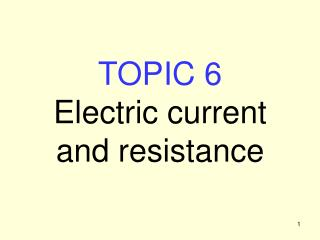DownloadDownload PresentationTOPIC 6 Electric current and resistance

# TOPIC 6 Electric current and resistance

Télécharger la présentation## TOPIC 6 Electric current and resistance

- - - - - - - - - - - - - - - - - - - - - - - - - - - E N D - - - - - - - - - - - - - - - - - - - - - - - - - - -
##### Presentation Transcript

1. TOPIC 6Electric currentand resistance

2. Electrons in Conductors • Conductors have free electrons, which • Are in continuous rapid motion – thermal and quantum effects • Undergo frequent scattering from the crystal lattice (positive ions) • Random motion does not constitute a current • An applied electric field results in a small drift velocity superimposed on the random motion • This drift gives a net movement of charge – an electric current, I

3. Electric Current Unit of current is Amp (Ampère), 1 A = 1 C s–1 Continuous current through conductor  potential difference between ends – eg due to battery Battery raises positive charges from low potential (negative terminal) to high potential (positive terminal) As much charge enters one end of the conductor (eg wire) as leaves at the other end – it does not charge up! Current only flows in a closed loop or circuit Current density J = current flow per unit area (perpendicular to current) J = I/A

4. Electrical Resistance The current I flowing through a component depends on the potential difference between its ends, V. We can define the resistance R of the component from Unit of resistance : ohm  (1  = 1 volt per amp) Conductance G = 1 / R (units –1 or mho)

5. Drude Model Assume n electrons (of charge q = –e) per unit volume Applied electric field E gives acceleration Mean time between collisions with lattice =  Drift velocity (superimposed on random motion) Charge Q in dotted cylinder = n Aq, passes end plane in time t = /vd, so I = nAqvd Hence

6. Resistivity & Conductivity • Drude model  • We can write this  is the resistivity, with units  m • = 1 /  = conductivity Note J = I/ A V = E 

7. Example 1 – Resistance, drift velocity Copper has a resistivity of 1.710–8  m, and 8.5 1028 m–3 free electrons. What is the mean time between collisions between a conduction electron and the lattice? What will the drift velocity be when 2 V is applied across a 5 m sample of copper? What resistance will a copper coil have if it is formed of 1000 turns of wire, of diameter 1 mm, wrapped around a tube of radius 3 cm?

8. Temperature Coefficient of Resistivity Increased temperature  increased lattice vibrations  increased electron scattering  increased resistivity in most materials Approximation for modest temperature changes: Here  is the temperature coefficient of resistivity. Example 2: A sample of platinum has a resistance of 30.00 at 20C, and 39.41 at 100C. What is the coefficient of resistivity for platinum? What would the resistance be at 0C?

9. Electrical Power The energy loss of a charge Q falling through potential difference V is Q V. The power dissipated (rate of energy loss) is therefore P = dQ/dtV = I V. Using V = I R, this can be expressed in a variety of useful ways: Example 3 A resistance of 3 is connected across a potential difference of 2 V. What is the current which flows, and how much power is dissipated?